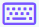Easy
Medium
Hard

## Second derivative calculator with steps

Second derivative calculator is used to calculate the 2nd-order differential of the function. It calculates the second-order derivative by differentiating the function twice.

A double derivative calculator provides a step-by-step solution. It calculates the first derivative and then the second derivative of the functions.

## How does 2nd derivative calculator work?

Follow the below steps to use this second derivative calculator.

• Enter the function.
• Select the variable.
• Use the keypad iconto enter the mathematical symbols.
• Press the calculate button.
• Click the reset button to solve another function.

## What is the second derivative?

The process of finding the differential of a function with respect to its variable is known as differentiation.

Similarly, the process of finding the second-order derivative of a similar function is known as a second differentiation. It is often called a second-order derivative.

The second-order derivative is a technique that calculates the derivative of the first differential function.

The notation for the second differential is $$\frac{d^2}{dx^2}$$ or $$f''\left(x\right)$$.

## How to calculate the second derivative?

Following are a few examples solved by our second derivative test calculator.

Example 1

Find the second derivative of $$4x^2+cos\left(x\right)$$?

Solution

Step 1: Apply the differential notation on the given function.

$$\frac{d}{dx}\left(4x^2+cos\left(x\right)\right)$$

Step 2: According to sum rule, apply the differential notation separately.

$$\frac{d}{dx}\left(4x^2+cos\left(x\right)\right)=\frac{d}{dx}\left(4x^2\right)+\frac{d}{dx}\left(cos\left(x\right)\right)$$

Step 3: Now apply the power rule and differentiate the above term.

$$\frac{d}{dx}\left(4x^2+cos\left(x\right)\right)=\left(4\cdot 2\right)x^{2-1}+\left(-sin\left(x\right)\right)$$

$$\frac{d}{dx}\left(4x^2+cos\left(x\right)\right)=8x-sin\left(x\right)$$

Step 4: Now apply the differential again on the above first derivative function to get the second order differential.

$$\frac{d}{dx}\left[\frac{d}{dx}\left(4x^2+cos\left(x\right)\right)\right]=\frac{d}{dx}\left[8x-sin\left(x\right)\right]$$

$$\frac{d^2}{dx^2}\left(4x^2+cos\left(x\right)\right)=\frac{d}{dx}\left[8x-sin\left(x\right)\right]$$

Step 5: Apply the difference rule and differentiate the function.

$$\frac{d^2}{dx^2}\left(4x^2+cos\left(x\right)\right)=\frac{d}{dx}\left(8x\right)-\frac{d}{dx}\left(sin\left(x\right)\right)$$

$$\frac{d^2}{dx^2}\left(4x^2+cos\left(x\right)\right)=\left(8\left(1\right)\right)-\left(cos\left(x\right)\right)$$

$$\frac{d^2}{dx^2}\left(4x^2+cos\left(x\right)\right)=8-cos\left(x\right)$$

Example 2

Find the second derivative of $$xsin\left(x\right)+32$$?

Solution

Step 1: Apply the differential notation on the given function.

$$\frac{d}{dx}\left(xsin\left(x\right)+32\right)$$

Step 2: According to sum rule, apply the differential notation separately.

$$\frac{d}{dx}\left(xsin\left(x\right)+32\right)=\frac{d}{dx}\left(xsin\left(x\right)\right)+\frac{d}{dx}\left(32\right)$$

Step 3: Now differentiate the above term.

$$\frac{d}{dx}\left(xsin\left(x\right)+32\right)=sin\left(x\right)\frac{d}{dx}\left(x\right)+x\frac{d}{dx}\left(sin\left(x\right)\right)+\frac{d}{dx}\left(32\right)$$

$$\frac{d}{dx}\left(xsin\left(x\right)+32\right)=sin\left(x\right)\left(1\right)+x\left(cos\left(x\right)\right)+\left(0\right)$$

$$\frac{d}{dx}\left(xsin\left(x\right)+32\right)=sin\left(x\right)+xcos\left(x\right)$$

Step 4: Now apply the differential again on the above first derivative function to get the second order differential.

$$\frac{d}{dx}\left[\frac{d}{dx}\left(xsin\left(x\right)+32\right)\right]=\frac{d}{dx}\left[sin\left(x\right)+xcos\left(x\right)\right]$$

$$\frac{d^2}{dx^2}\left(xsin\left(x\right)+32\right)=\frac{d}{dx}\left[sin\left(x\right)+xcos\left(x\right)\right]$$

Step 5: Apply the sum and product rules and differentiate the function.

$$\frac{d^2}{dx^2}\left(xsin\left(x\right)+32\right)=\frac{d}{dx}\left(sin\left(x\right)\right)+\frac{d}{dx}\left(xcos\left(x\right)\right)$$

$$\frac{d^2}{dx^2}\left(xsin\left(x\right)+32\right)=\frac{d}{dx}\left(sin\left(x\right)\right)+cos\left(x\right)\frac{d}{dx}\left(x\right)+x\frac{d}{dx}\left(cos\left(x\right)\right)$$

$$\frac{d^2}{dx^2}\left(xsin\left(x\right)+32\right)=\left(cos\left(x\right)\right)+cos\left(x\right)\left(1\right)+x\left(-sin\left(x\right)\right)$$

$$\frac{d^2}{dx^2}\left(xsin\left(x\right)+32\right)=cos\left(x\right)+cos\left(x\right)-xsin\left(x\right)$$

$$\frac{d^2}{dx^2}\left(xsin\left(x\right)+32\right)=2cos\left(x\right)-xsin\left(x\right)$$

## Table of some 2nd derivatives of functions

Following are a few examples of the second differential solved by our second differential calculator.

 Second derivative of Output sinx -sinx e^x e^x sqrt(x) $$\frac{-1}{\:4x^{\frac{3}{2}}}$$ 1/x $$\frac{2}{\:x^3}$$ a^x $$a^xlog^2\left(a\right)$$

### Resources

What is Second derivative? | Wikipedia.

Use android or iOS app of our limit calculator on your mobile